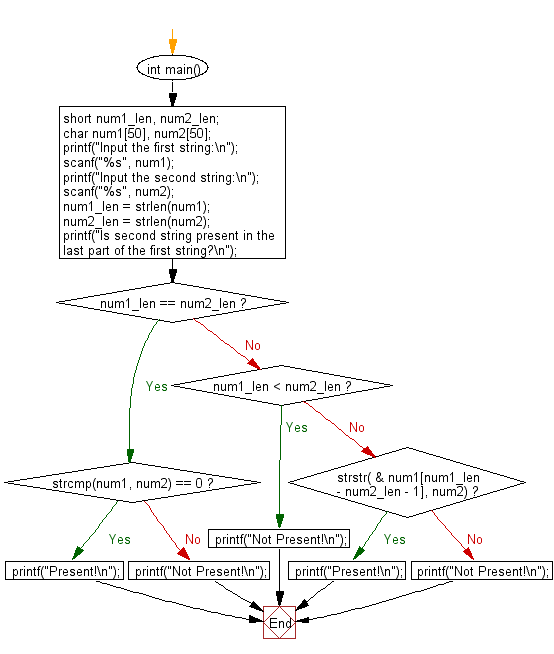﻿ C : Check if a string appears at the end of another string

# C Exercises: Check if a string is present in the last part of another string

## C Basic Declarations and Expressions: Exercise-131 with Solution

Write a C program that accepts two strings and checks whether the second string is present in the last part of the first string.

Sample Solution:

C Code:

``````#include <stdio.h>
#include <string.h>

int main() {
short num1_len, num2_len;
char num1, num2;

// Prompt the user to input the first string
printf("Input the first string:\n");
scanf("%s", num1);

// Prompt the user to input the second string
printf("Input the second string:\n");
scanf("%s", num2);

// Get the lengths of the two strings
num1_len = strlen(num1);
num2_len = strlen(num2);

// Check if the second string is present in the last part of the first string
printf("Is second string present in the last part of the first string?\n");

if (num1_len == num2_len) // If the lengths are equal
if (strcmp(num1, num2) == 0) // If the strings are equal
printf("Present!\n");
else
printf("Not Present!\n");
else if (num1_len < num2_len) // If the first string is shorter than the second
printf("Not Present!\n");
else if (strstr(&num1[num1_len - num2_len - 1], num2)) // If the second string is found in the last part of the first string
printf("Present!\n");
else
printf("Not Present!\n");

return 0; // End of program
}
``````

Sample Output:

```Input the first string:
abcdef
Input the second string:
ef
Is second string present in the last part of the first string?
Present!
```

Flowchart:C programming Code Editor:

What is the difficulty level of this exercise?

Test your Programming skills with w3resource's quiz.

﻿